# Chapter 13 Overview

TODO: Move current content into the following placeholder chapters. Add details.

## 13.1 Methods

### 13.1.1 Principal Component Analysis

To perform PCA in R we will use prcomp(). See ?prcomp() for details.

### 13.1.2$$k$$-Means Clustering

To perform $$k$$-means in R we will use kmeans(). See ?kmeans() for details.

### 13.1.3 Hierarchical Clustering

To perform hierarchical clustering in R we will use hclust(). See ?hclust() for details.

## 13.2 Examples

### 13.2.1 US Arrests

library(ISLR)
data(USArrests)
apply(USArrests, 2, mean)
##   Murder  Assault UrbanPop     Rape
##    7.788  170.760   65.540   21.232
apply(USArrests, 2, sd)
##    Murder   Assault  UrbanPop      Rape
##  4.355510 83.337661 14.474763  9.366385

“Before” performing PCA, we will scale the data. (This will actually happen inside the prcomp() function.)

USArrests_pca = prcomp(USArrests, scale = TRUE)

A large amount of information is stored in the output of prcomp(), some of which can neatly be displayed with summary().

names(USArrests_pca)
##  "sdev"     "rotation" "center"   "scale"    "x"
summary(USArrests_pca)
## Importance of components:
##                           PC1    PC2     PC3     PC4
## Standard deviation     1.5749 0.9949 0.59713 0.41645
## Proportion of Variance 0.6201 0.2474 0.08914 0.04336
## Cumulative Proportion  0.6201 0.8675 0.95664 1.00000
USArrests_pca$center ## Murder Assault UrbanPop Rape ## 7.788 170.760 65.540 21.232 USArrests_pca$scale
##    Murder   Assault  UrbanPop      Rape
##  4.355510 83.337661 14.474763  9.366385
USArrests_pca$rotation ## PC1 PC2 PC3 PC4 ## Murder -0.5358995 0.4181809 -0.3412327 0.64922780 ## Assault -0.5831836 0.1879856 -0.2681484 -0.74340748 ## UrbanPop -0.2781909 -0.8728062 -0.3780158 0.13387773 ## Rape -0.5434321 -0.1673186 0.8177779 0.08902432 We see that $center and $scale give the mean and standard deviations for the original variables. $rotation gives the loading vectors that are used to rotate the original data to obtain the principal components.

dim(USArrests_pca$x) ##  50 4 dim(USArrests) ##  50 4 head(USArrests_pca$x)
##                   PC1        PC2         PC3          PC4
## Alabama    -0.9756604  1.1220012 -0.43980366  0.154696581
## Alaska     -1.9305379  1.0624269  2.01950027 -0.434175454
## Arizona    -1.7454429 -0.7384595  0.05423025 -0.826264240
## Arkansas    0.1399989  1.1085423  0.11342217 -0.180973554
## California -2.4986128 -1.5274267  0.59254100 -0.338559240
## Colorado   -1.4993407 -0.9776297  1.08400162  0.001450164

The dimension of the principal components is the same as the original data. The principal components are stored in $x. scale(as.matrix(USArrests))[1, ] %*% USArrests_pca$rotation[, 1]
##            [,1]
## [1,] -0.9756604
scale(as.matrix(USArrests))[1, ] %*% USArrests_pca$rotation[, 2] ## [,1] ## [1,] 1.122001 scale(as.matrix(USArrests))[1, ] %*% USArrests_pca$rotation[, 3]
##            [,1]
## [1,] -0.4398037
scale(as.matrix(USArrests))[1, ] %*% USArrests_pca$rotation[, 4] ## [,1] ## [1,] 0.1546966 head(scale(as.matrix(USArrests)) %*% USArrests_pca$rotation[,1])
##                  [,1]
## Alabama    -0.9756604
## Arizona    -1.7454429
## Arkansas    0.1399989
## California -2.4986128
## Colorado   -1.4993407
head(USArrests_pca$x[, 1]) ## Alabama Alaska Arizona Arkansas California Colorado ## -0.9756604 -1.9305379 -1.7454429 0.1399989 -2.4986128 -1.4993407 sum(USArrests_pca$rotation[, 1] ^ 2)
##  1
USArrests_pca$rotation[, 1] %*% USArrests_pca$rotation[, 2]
##               [,1]
## [1,] -1.665335e-16
USArrests_pca$rotation[, 1] %*% USArrests_pca$rotation[, 3]
##              [,1]
## [1,] 1.110223e-16
USArrests_pca$x[, 1] %*% USArrests_pca$x[, 2]
##               [,1]
## [1,] -7.938095e-15
USArrests_pca$x[, 1] %*% USArrests_pca$x[, 3]
##              [,1]
## [1,] 1.787459e-14

The above verifies some of the “math” of PCA. We see how the loadings obtain the principal components from the original data. We check that the loading vectors are normalized. We also check for orthogonality of both the loading vectors and the principal components. (Note the above inner products aren’t exactly 0, but that is simply a numerical issue.)

biplot(USArrests_pca, scale = 0, cex = 0.5)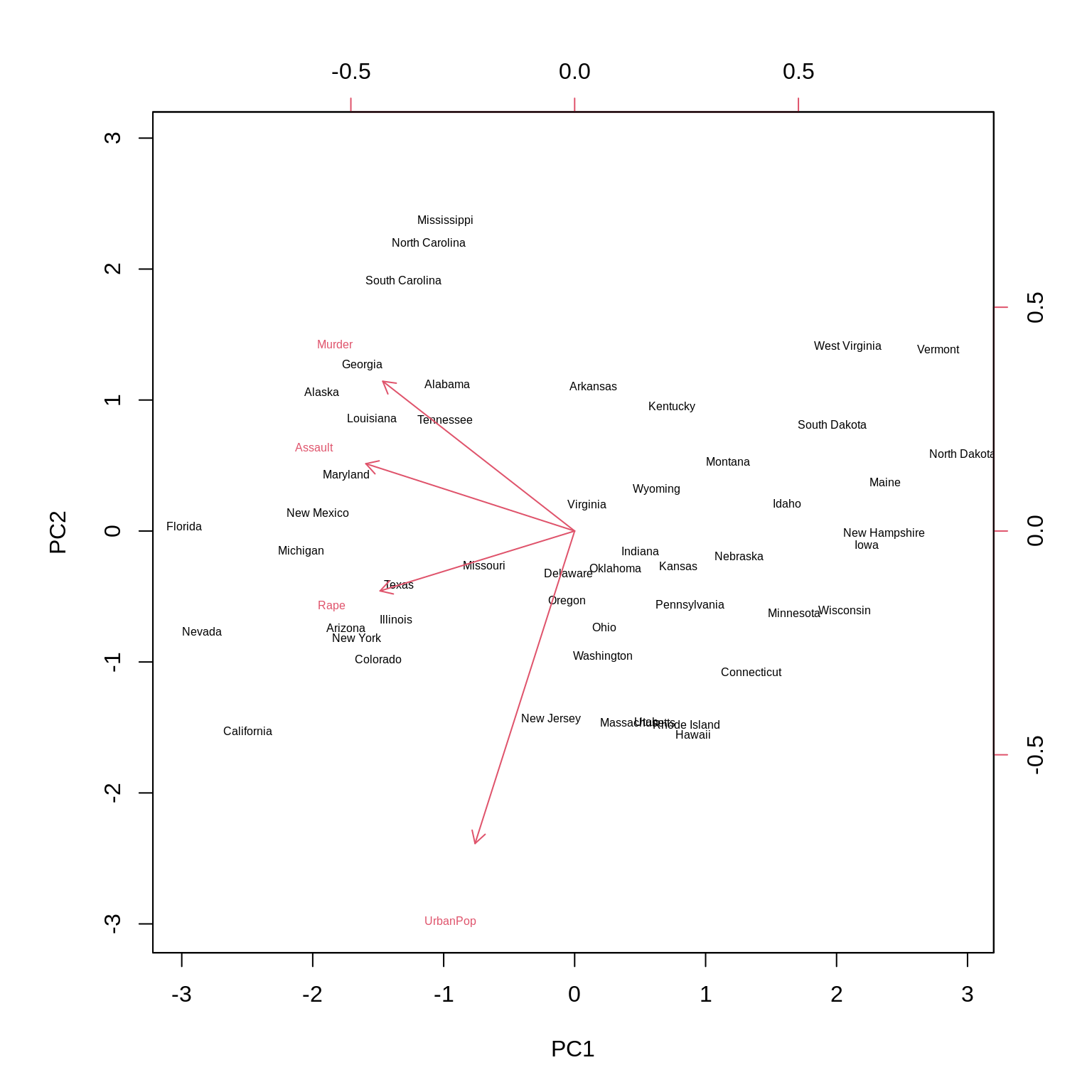A biplot can be used to visualize both the principal component scores and the principal component loadings. (Note the two scales for each axis.)

USArrests_pca$sdev ##  1.5748783 0.9948694 0.5971291 0.4164494 USArrests_pca$sdev ^ 2 / sum(USArrests_pca$sdev ^ 2) ##  0.62006039 0.24744129 0.08914080 0.04335752 Frequently we will be interested in the proportion of variance explained by a principal component. get_PVE = function(pca_out) { pca_out$sdev ^ 2 / sum(pca_out$sdev ^ 2) } So frequently, we would be smart to write a function to do so. pve = get_PVE(USArrests_pca) pve ##  0.62006039 0.24744129 0.08914080 0.04335752 plot( pve, xlab = "Principal Component", ylab = "Proportion of Variance Explained", ylim = c(0, 1), type = 'b' )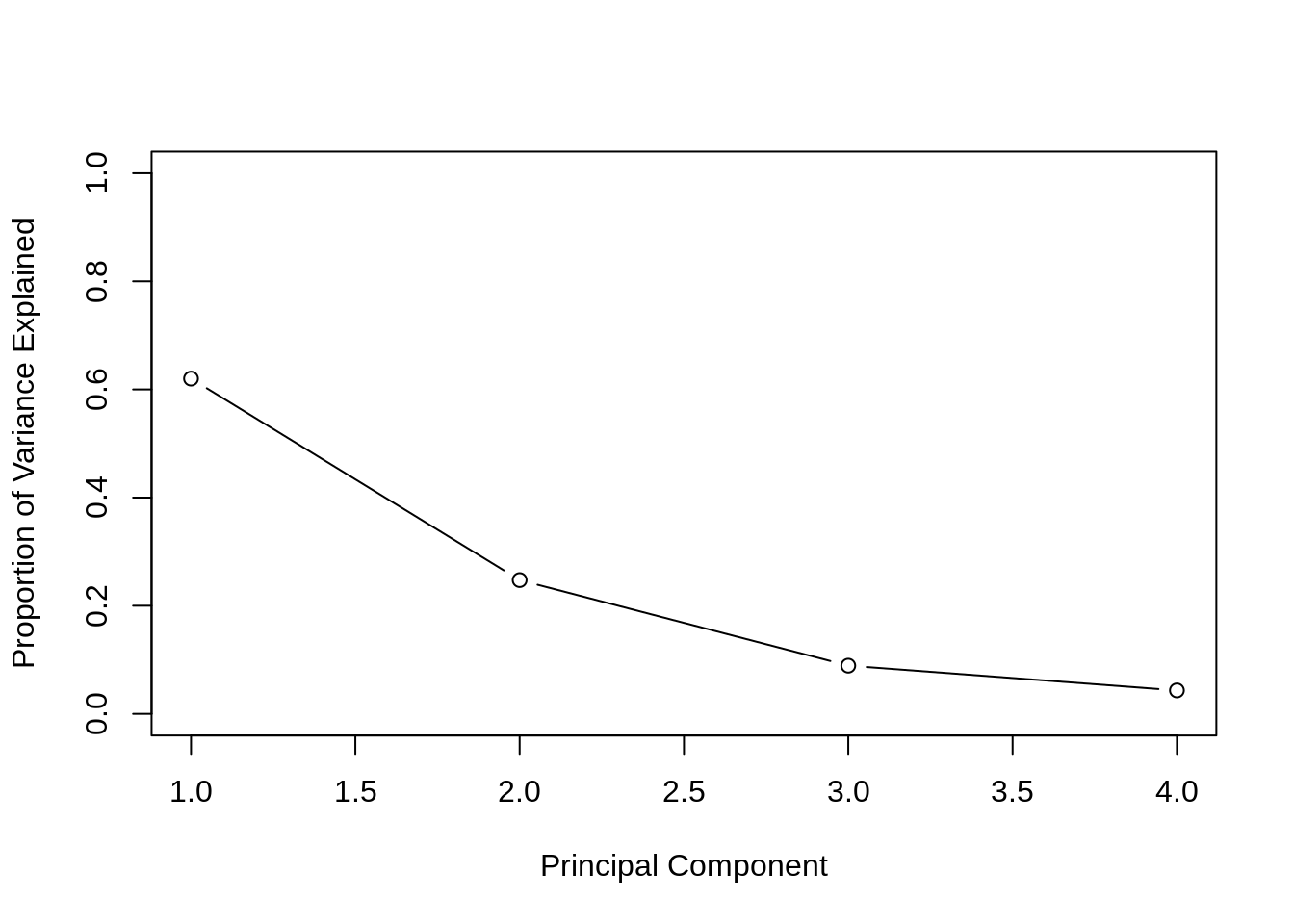We can then plot the proportion of variance explained for each PC. As expected, we see the PVE decrease. cumsum(pve) ##  0.6200604 0.8675017 0.9566425 1.0000000 plot( cumsum(pve), xlab = "Principal Component", ylab = "Cumulative Proportion of Variance Explained", ylim = c(0, 1), type = 'b' )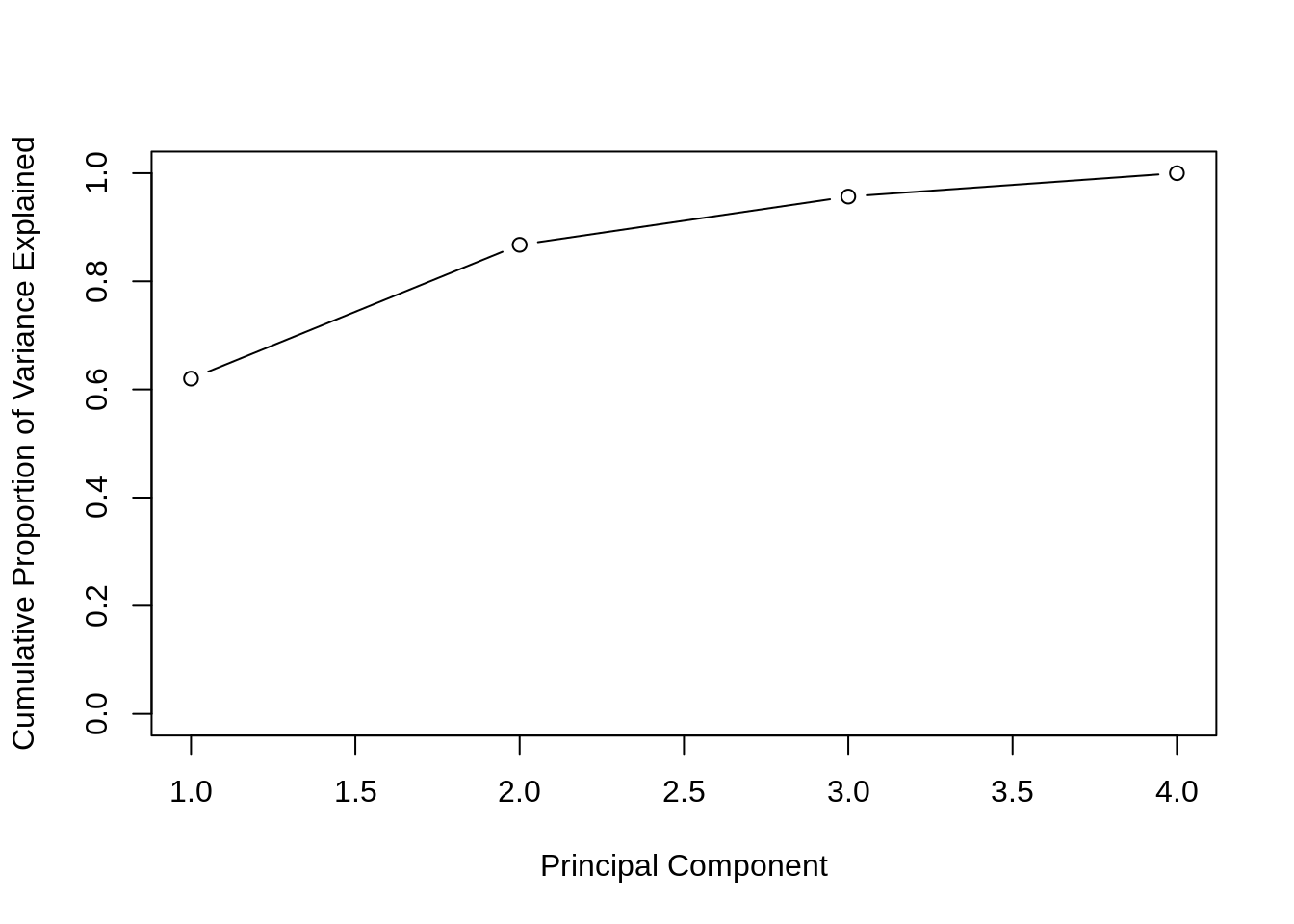Often we are interested in the cumulative proportion. A common use of PCA outside of visualization is dimension reduction for modeling. If $$p$$ is large, PCA is performed, and the principal components that account for a large proportion of variation, say 95%, are used for further analysis. In certain situations that can reduce the dimensionality of data significantly. This can be done almost automatically using caret: library(caret) library(mlbench) data(Sonar) set.seed(18) using_pca = train(Class ~ ., data = Sonar, method = "knn", trControl = trainControl(method = "cv", number = 5), preProcess = "pca", tuneGrid = expand.grid(k = c(1, 3, 5, 7, 9))) regular_scaling = train(Class ~ ., data = Sonar, method = "knn", trControl = trainControl(method = "cv", number = 5), preProcess = c("center", "scale"), tuneGrid = expand.grid(k = c(1, 3, 5, 7, 9))) max(using_pca$results$Accuracy) ##  0.8513357 max(regular_scaling$results$Accuracy) ##  0.8267131 using_pca$preProcess
## Created from 208 samples and 60 variables
##
## Pre-processing:
##   - centered (60)
##   - ignored (0)
##   - principal component signal extraction (60)
##   - scaled (60)
##
## PCA needed 30 components to capture 95 percent of the variance

It won’t always outperform simply using the original predictors, but here using 30 of 60 principal components shows a slight advantage over using all 60 predictors. In other situation, it may result in a large perform gain.

### 13.2.2 Simulated Data

library(MASS)
set.seed(42)
n = 180
p = 10
clust_data = rbind(
mvrnorm(n = n / 3, sample(c(1, 2, 3, 4), p, replace = TRUE), diag(p)),
mvrnorm(n = n / 3, sample(c(1, 2, 3, 4), p, replace = TRUE), diag(p)),
mvrnorm(n = n / 3, sample(c(1, 2, 3, 4), p, replace = TRUE), diag(p))
)

Above we simulate data for clustering. Note that, we did this in a way that will result in three clusters.

true_clusters = c(rep(3, n / 3), rep(1, n / 3), rep(2, n / 3))

We label the true clusters 1, 2, and 3 in a way that will “match” output from $$k$$-means. (Which is somewhat arbitrary.)

kmean_out = kmeans(clust_data, centers = 3, nstart = 10)
names(kmean_out)
##  "cluster"      "centers"      "totss"        "withinss"     "tot.withinss"
##  "betweenss"    "size"         "iter"         "ifault"

Notice that we used nstart = 10 which will give us a more stable solution by attempting 10 random starting positions for the means. Also notice we chose to use centers = 3. (The $$k$$ in $$k$$-mean). How did we know to do this? We’ll find out on the homework. (It will involve looking at tot.withinss)

kmean_out
## K-means clustering with 3 clusters of sizes 60, 57, 63
##
## Cluster means:
##        [,1]     [,2]      [,3]     [,4]     [,5]     [,6]     [,7]     [,8]
## 1 0.9546789 1.058535 0.9343691 1.050995 1.863093 4.045362 1.949864 1.923591
## 2 3.9349713 3.901193 2.1648511 1.029045 3.024529 3.917283 1.017899 3.858956
## 3 4.1010688 4.049770 3.8473862 2.103797 2.088527 3.209953 1.750652 1.874236
##        [,9]    [,10]
## 1 1.0041300 3.954435
## 2 1.1017062 1.932706
## 3 0.7874858 3.980819
##
## Clustering vector:
##    1 1 1 1 1 1 1 1 1 1 1 1 1 1 1 1 1 1 1 1 1 1 1 1 1 1 1 1 1 1 1 1 1 1 1 1 1
##   1 1 1 1 1 1 1 1 1 1 1 1 1 1 1 1 1 1 1 1 1 1 1 3 3 3 3 3 3 3 3 3 3 3 3 3 3
##   3 3 3 3 3 3 3 3 3 3 3 3 3 3 3 3 3 3 3 3 2 3 3 3 3 3 3 3 3 3 3 3 3 2 3 3 3
##  3 3 3 3 3 3 3 3 3 2 2 2 2 2 2 2 2 3 2 2 2 2 2 2 2 2 2 2 2 2 2 2 2 2 3 2 2
##  2 2 2 2 3 2 3 2 2 2 2 2 2 2 2 2 2 2 2 2 2 2 2 2 2 2 3 2 2 2 2 2
##
## Within cluster sum of squares by cluster:
##  580.8431 534.3726 634.7403
##  (between_SS / total_SS =  45.1 %)
##
## Available components:
##
##  "cluster"      "centers"      "totss"        "withinss"     "tot.withinss"
##  "betweenss"    "size"         "iter"         "ifault"
kmeans_clusters = kmean_out$cluster table(true_clusters, kmeans_clusters) ## kmeans_clusters ## true_clusters 1 2 3 ## 1 0 2 58 ## 2 0 55 5 ## 3 60 0 0 We check how well the clustering is working. dim(clust_data) ##  180 10 This data is “high dimensional” so it is difficult to visualize. (Anything more than 2 is hard to visualize.) plot( clust_data[, 1], clust_data[, 2], pch = 20, xlab = "First Variable", ylab = "Second Variable" )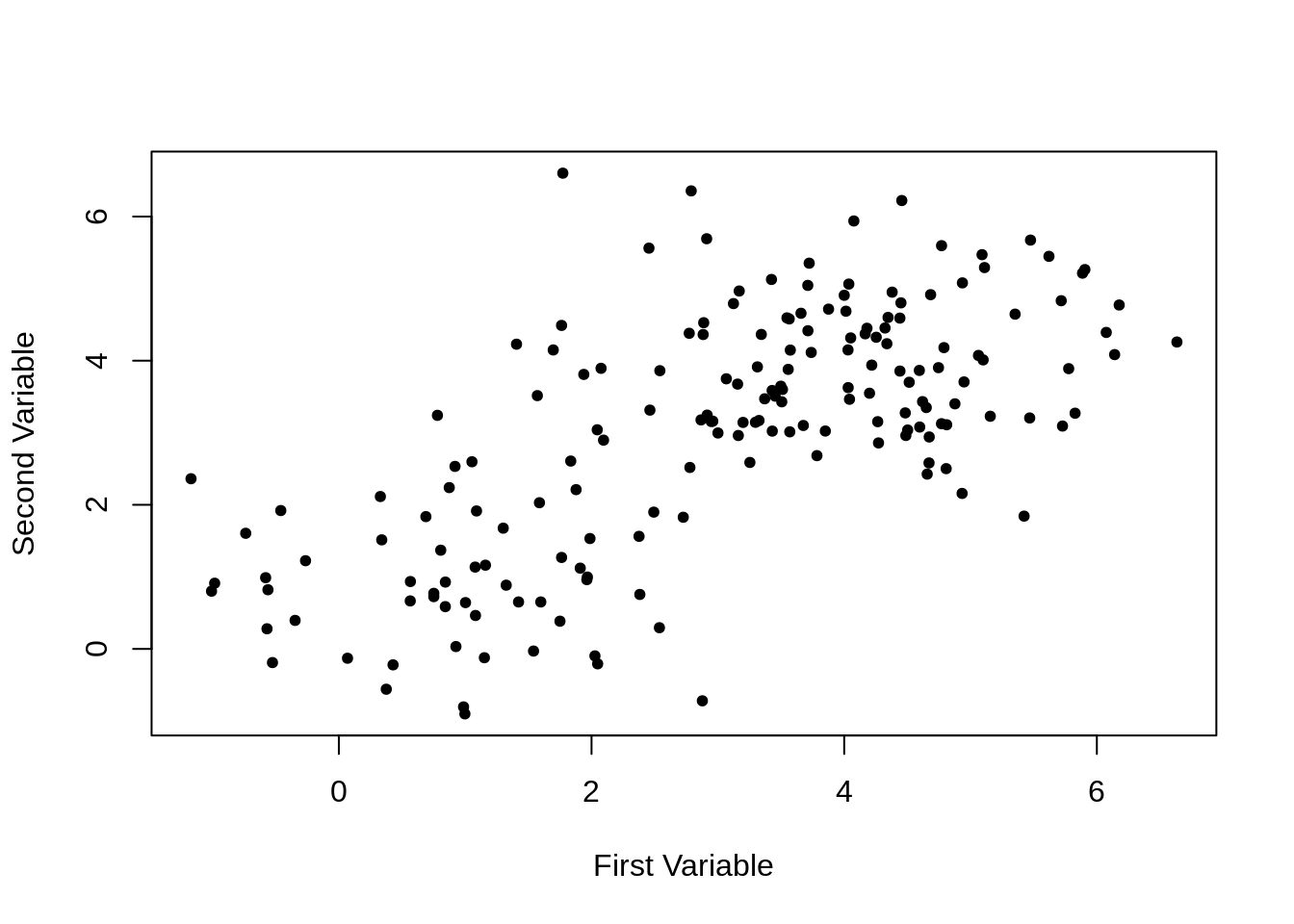Plotting the first and second variables simply results in a blob. plot( clust_data[, 1], clust_data[, 2], col = true_clusters, pch = 20, xlab = "First Variable", ylab = "Second Variable" )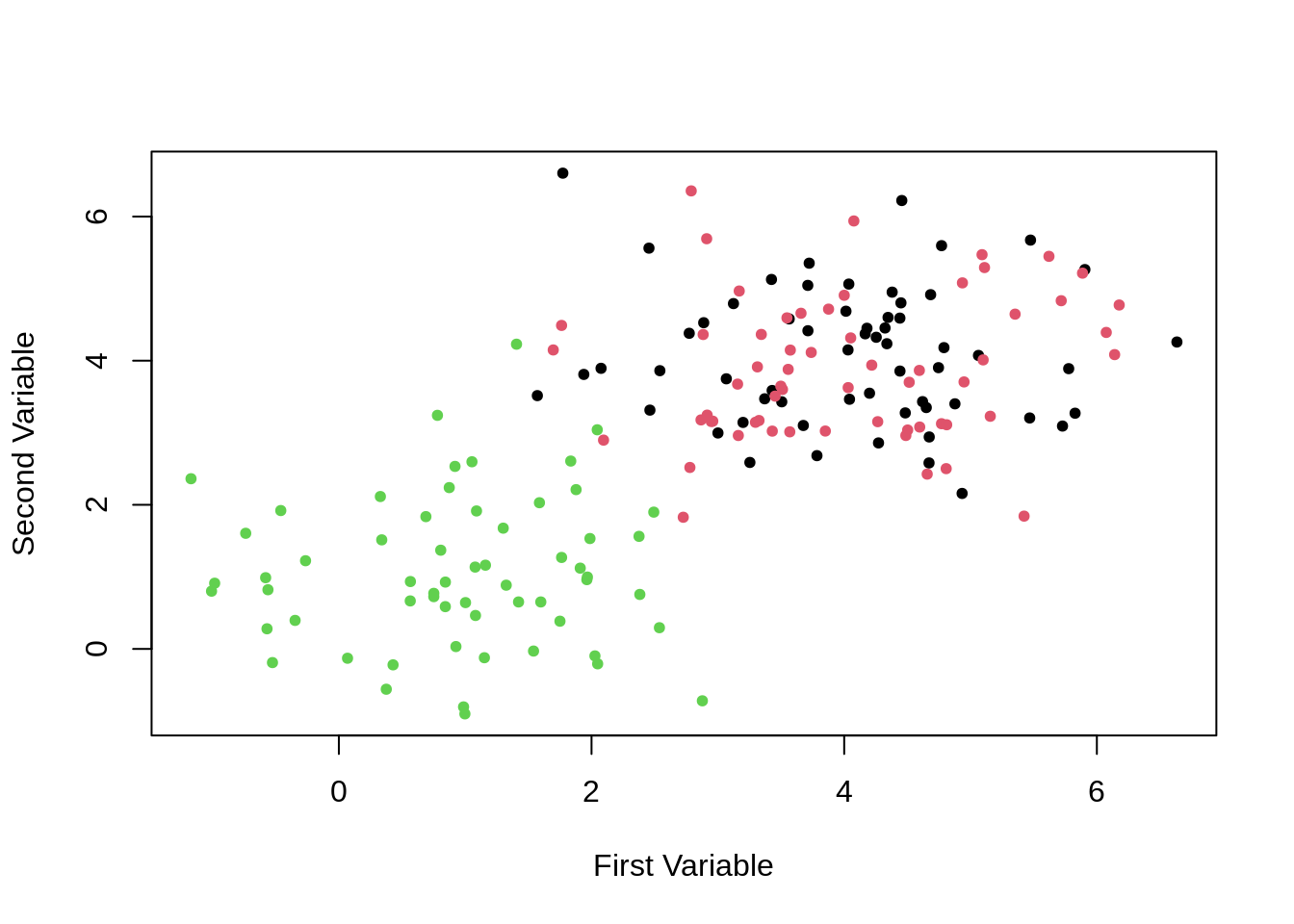Even when using their true clusters for coloring, this plot isn’t very helpful. clust_data_pca = prcomp(clust_data, scale = TRUE) plot( clust_data_pca$x[, 1],
clust_data_pca$x[, 2], pch = 0, xlab = "First Principal Component", ylab = "Second Principal Component" )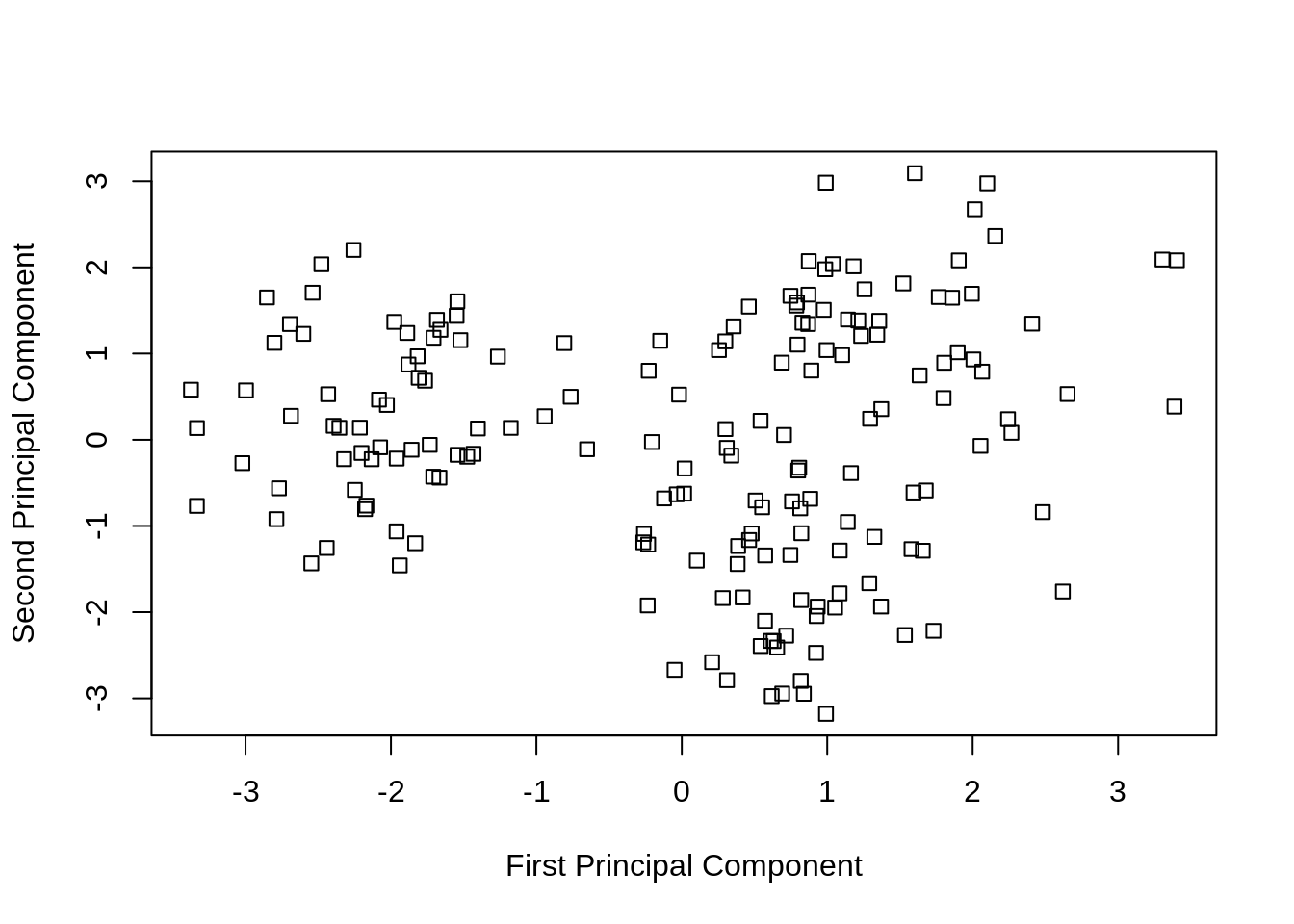If we instead plot the first two principal components, we see, even without coloring, one blob that is clearly separate from the rest. plot( clust_data_pca$x[, 1],
clust_data_pca$x[, 2], col = true_clusters, pch = 0, xlab = "First Principal Component", ylab = "Second Principal Component", cex = 2 ) points(clust_data_pca$x[, 1], clust_data_pca$x[, 2], col = kmeans_clusters, pch = 20, cex = 1.5)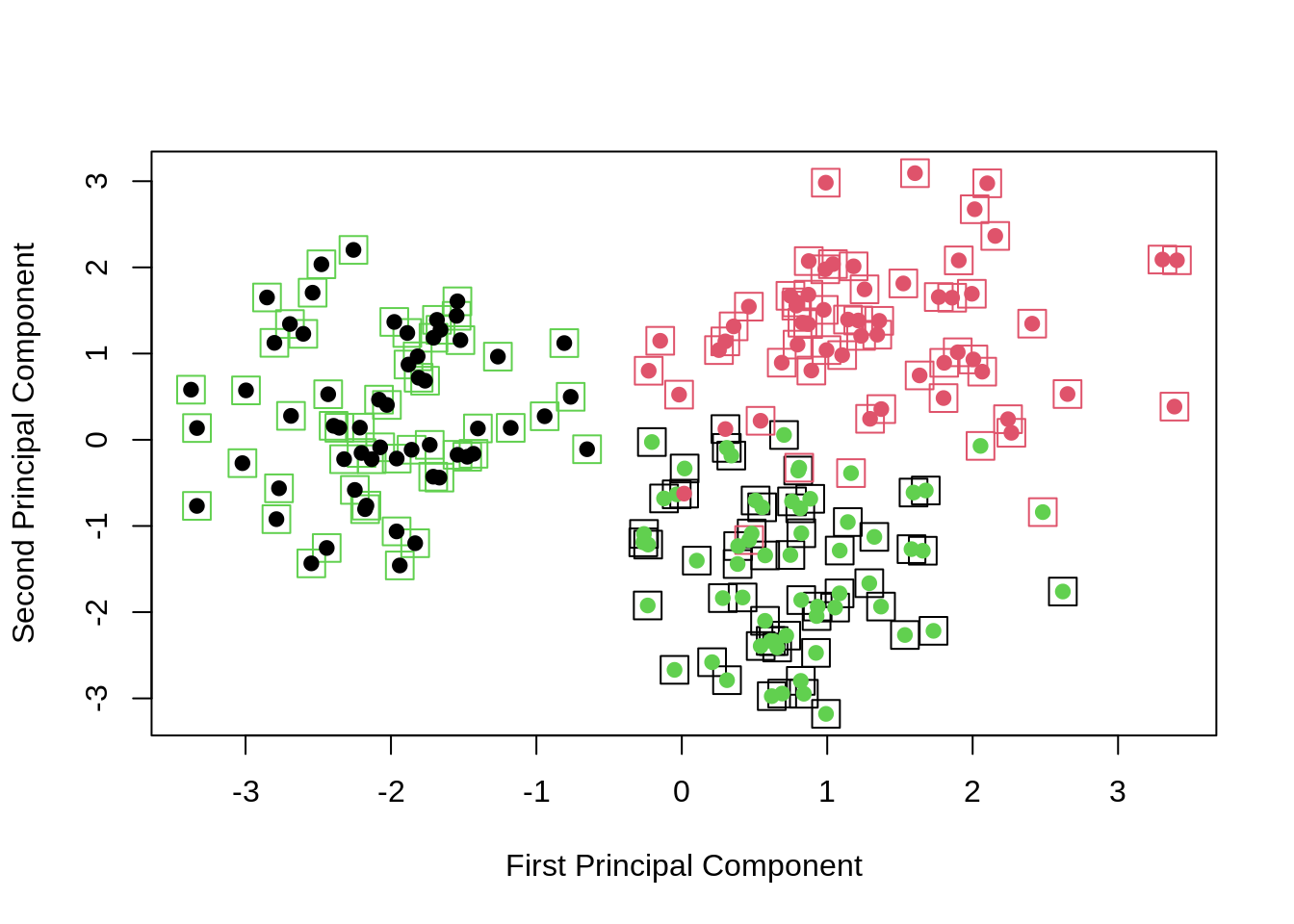Now adding the true colors (boxes) and the $$k$$-means results (circles), we obtain a nice visualization. clust_data_pve = get_PVE(clust_data_pca) plot( cumsum(clust_data_pve), xlab = "Principal Component", ylab = "Cumulative Proportion of Variance Explained", ylim = c(0, 1), type = 'b' )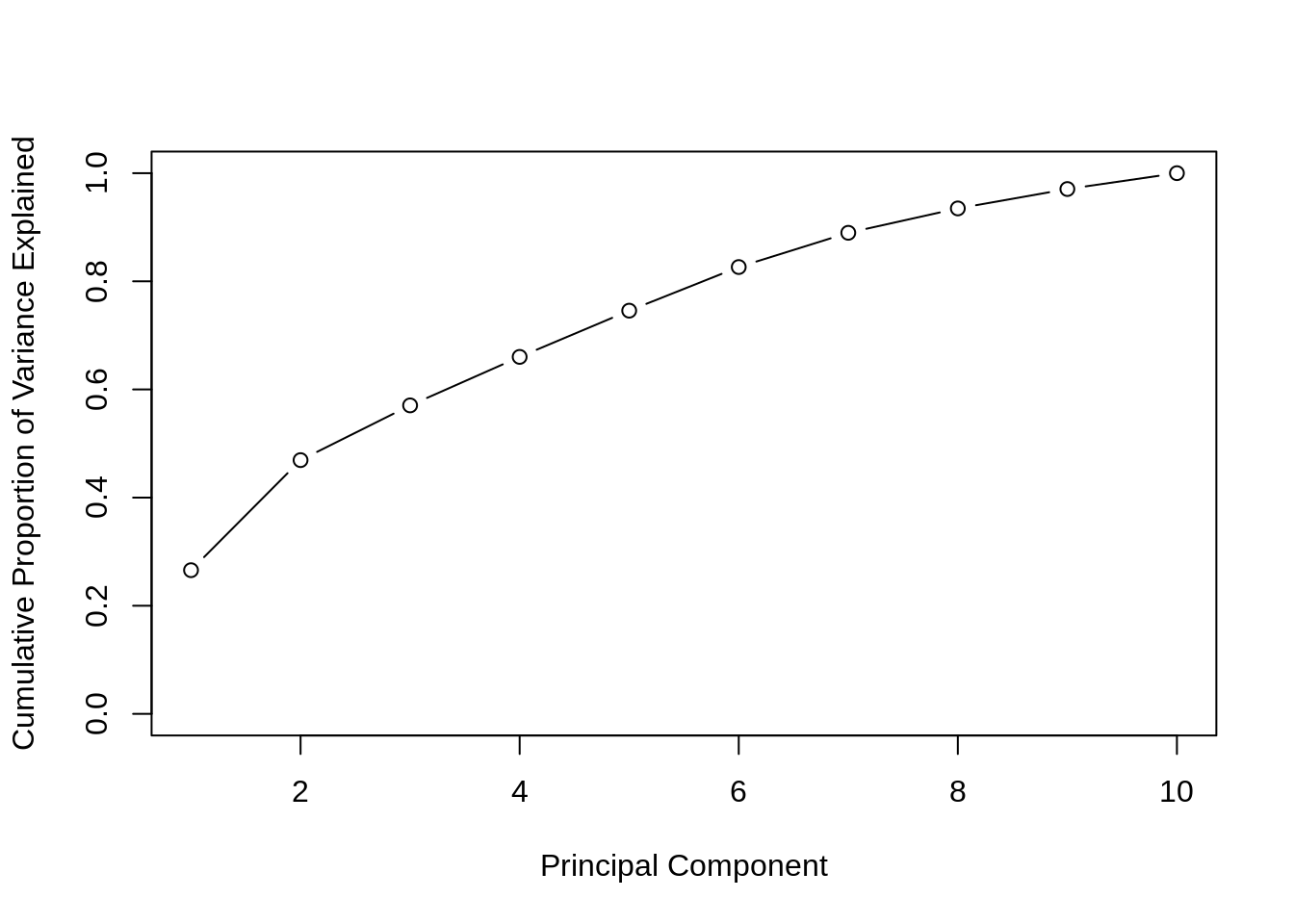The above visualization works well because the first two PCs explain a large proportion of the variance. #install.packages('sparcl') library(sparcl) To create colored dendrograms we will use ColorDendrogram() in the sparcl package. clust_data_hc = hclust(dist(scale(clust_data)), method = "complete") clust_data_cut = cutree(clust_data_hc , 3) ColorDendrogram(clust_data_hc, y = clust_data_cut, labels = names(clust_data_cut), main = "Simulated Data, Complete Linkage", branchlength = 1.5)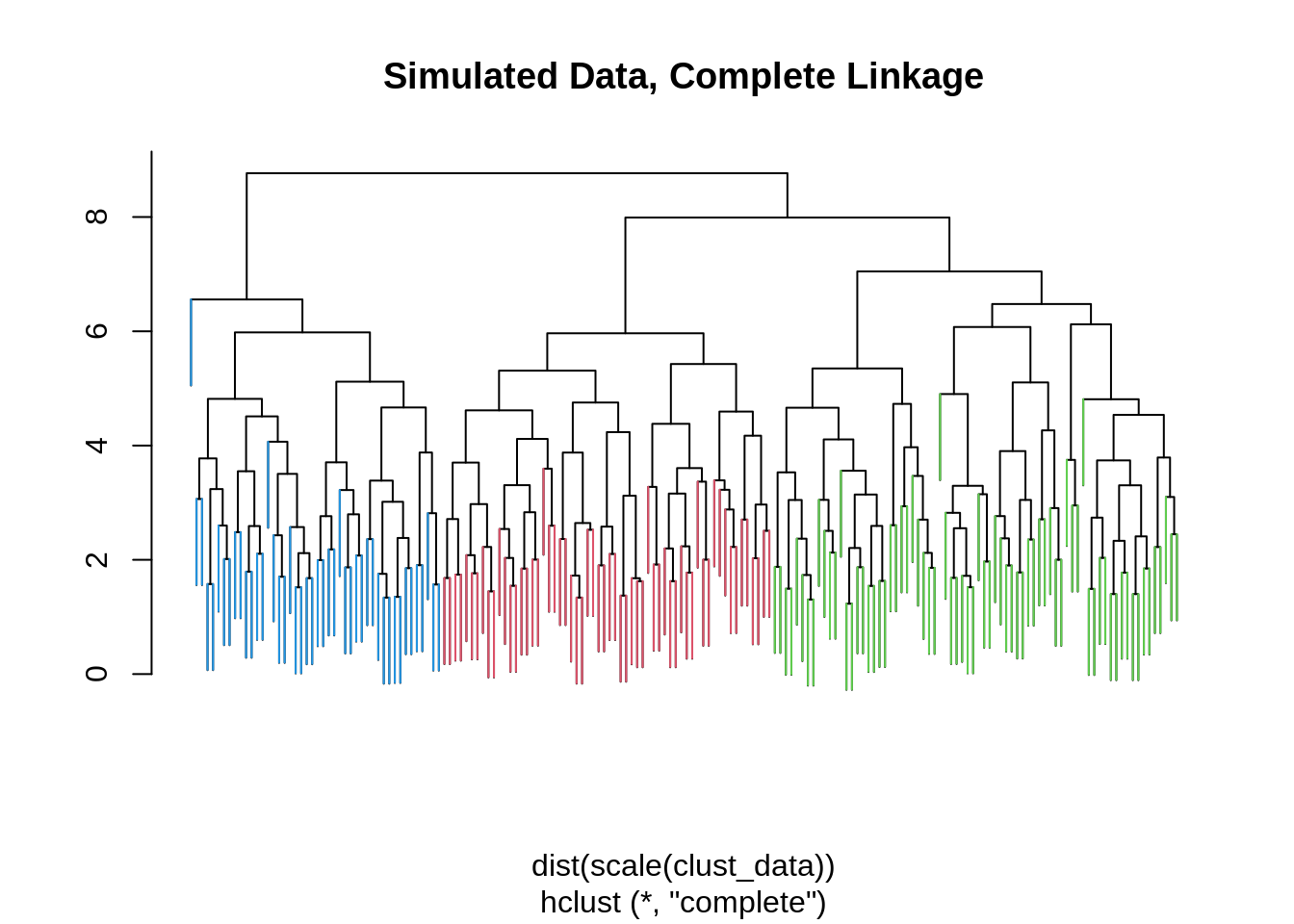Here we apply hierarchical clustering to the scaled data. The dist() function is used to calculate pairwise distances between the (scaled in this case) observations. We use complete linkage. We then use the cutree() function to cluster the data into 3 clusters. The ColorDendrogram() function is then used to plot the dendrogram. Note that the branchlength argument is somewhat arbitrary (the length of the colored bar) and will need to be modified for each dendrogram. table(true_clusters, clust_data_cut) ## clust_data_cut ## true_clusters 1 2 3 ## 1 0 16 44 ## 2 2 56 2 ## 3 58 2 0 We see in this case hierarchical clustering doesn’t “work” as well as $$k$$-means. clust_data_hc = hclust(dist(scale(clust_data)), method = "single") clust_data_cut = cutree(clust_data_hc , 3) ColorDendrogram(clust_data_hc, y = clust_data_cut, labels = names(clust_data_cut), main = "Simulated Data, Single Linkage", branchlength = 0.5)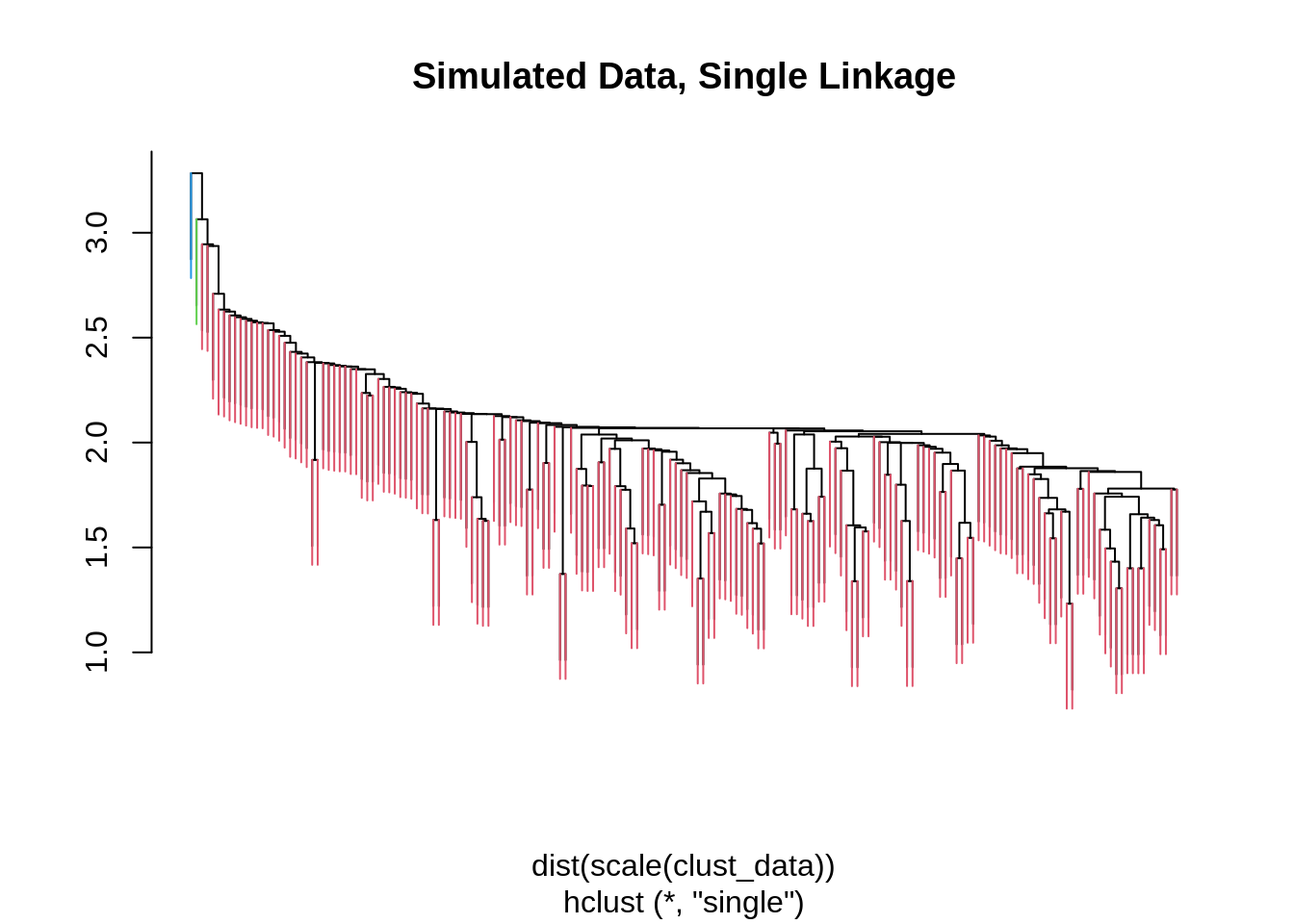table(true_clusters, clust_data_cut) ## clust_data_cut ## true_clusters 1 2 3 ## 1 59 1 0 ## 2 59 0 1 ## 3 60 0 0 clust_data_hc = hclust(dist(scale(clust_data)), method = "average") clust_data_cut = cutree(clust_data_hc , 3) ColorDendrogram(clust_data_hc, y = clust_data_cut, labels = names(clust_data_cut), main = "Simulated Data, Average Linkage", branchlength = 1)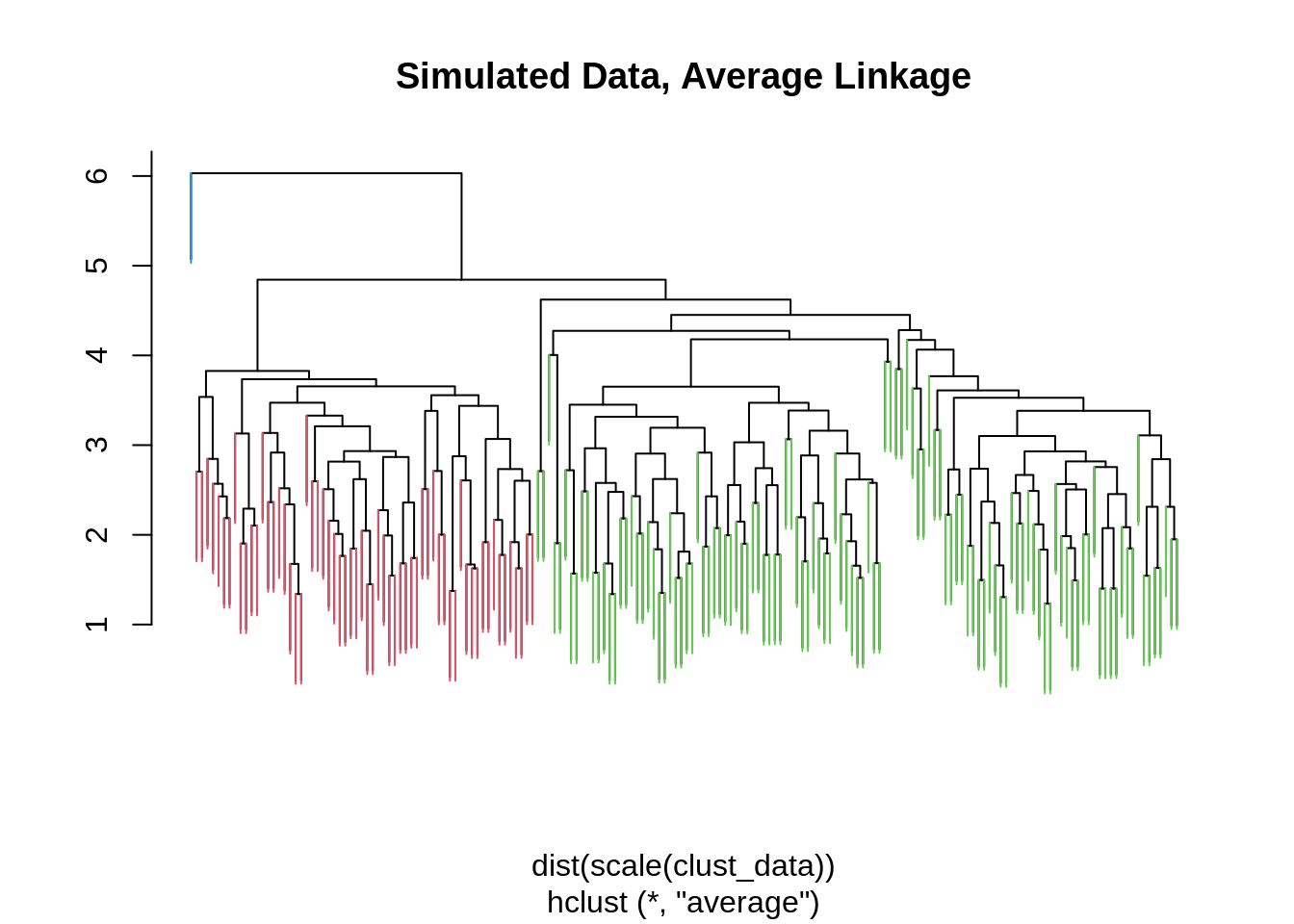table(true_clusters, clust_data_cut) ## clust_data_cut ## true_clusters 1 2 3 ## 1 1 58 1 ## 2 1 59 0 ## 3 60 0 0 We also try single and average linkage. Single linkage seems to perform poorly here, while average linkage seems to be working well. ### 13.2.3 Iris Data str(iris) ## 'data.frame': 150 obs. of 5 variables: ##$ Sepal.Length: num  5.1 4.9 4.7 4.6 5 5.4 4.6 5 4.4 4.9 ...
##  $Sepal.Width : num 3.5 3 3.2 3.1 3.6 3.9 3.4 3.4 2.9 3.1 ... ##$ Petal.Length: num  1.4 1.4 1.3 1.5 1.4 1.7 1.4 1.5 1.4 1.5 ...
##  $Petal.Width : num 0.2 0.2 0.2 0.2 0.2 0.4 0.3 0.2 0.2 0.1 ... ##$ Species     : Factor w/ 3 levels "setosa","versicolor",..: 1 1 1 1 1 1 1 1 1 1 ...
iris_pca = prcomp(iris[,-5], scale = TRUE)
iris_pca$rotation ## PC1 PC2 PC3 PC4 ## Sepal.Length 0.5210659 -0.37741762 0.7195664 0.2612863 ## Sepal.Width -0.2693474 -0.92329566 -0.2443818 -0.1235096 ## Petal.Length 0.5804131 -0.02449161 -0.1421264 -0.8014492 ## Petal.Width 0.5648565 -0.06694199 -0.6342727 0.5235971 lab_to_col = function (labels){ cols = rainbow (length(unique(labels))) cols[as.numeric (as.factor(labels))] } plot(iris_pca$x[,1], iris_pca$x[,2], col = lab_to_col(iris$Species), pch = 20)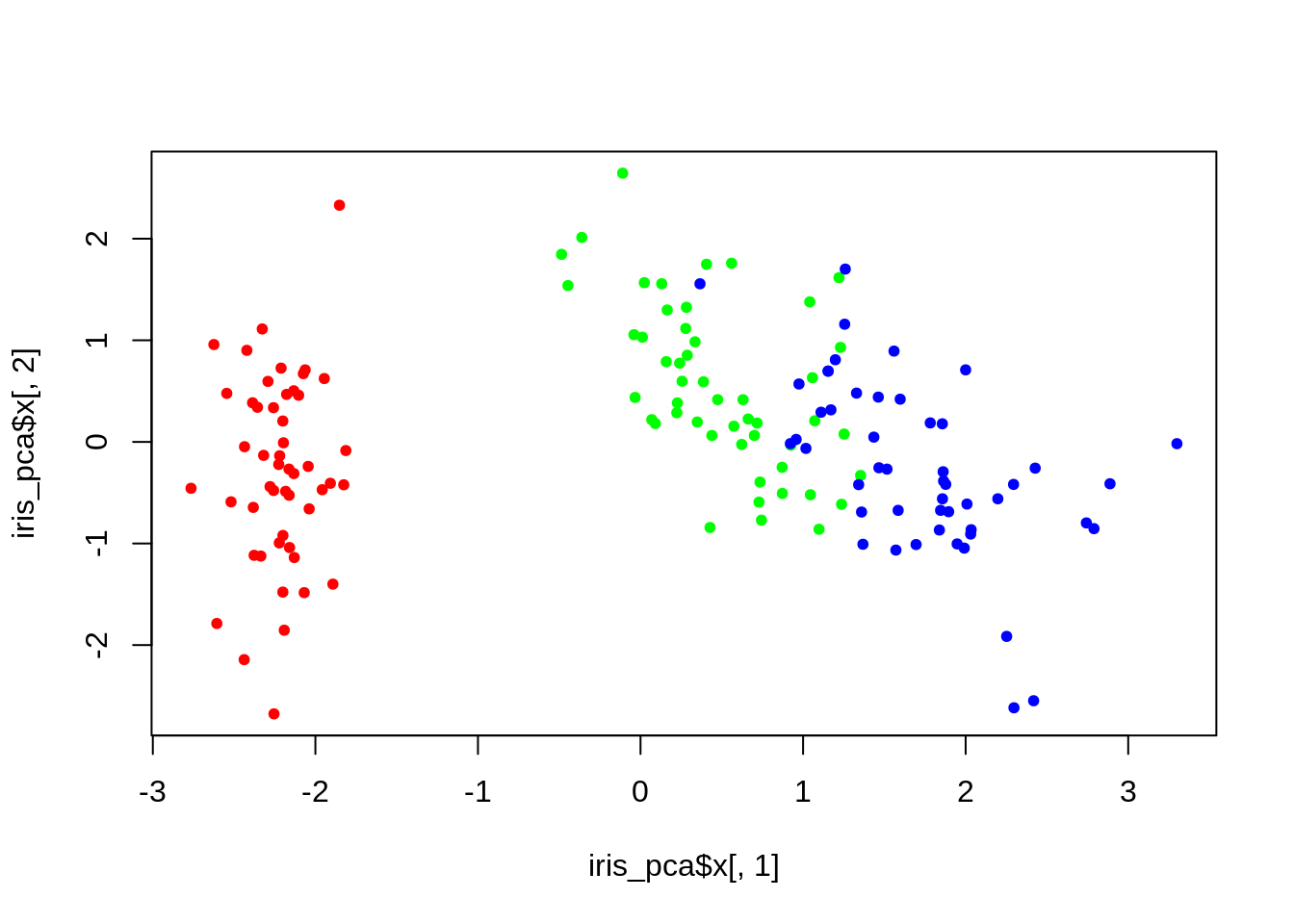plot(iris_pca$x[,3], iris_pca$x[,4], col = lab_to_col(iris$Species), pch = 20)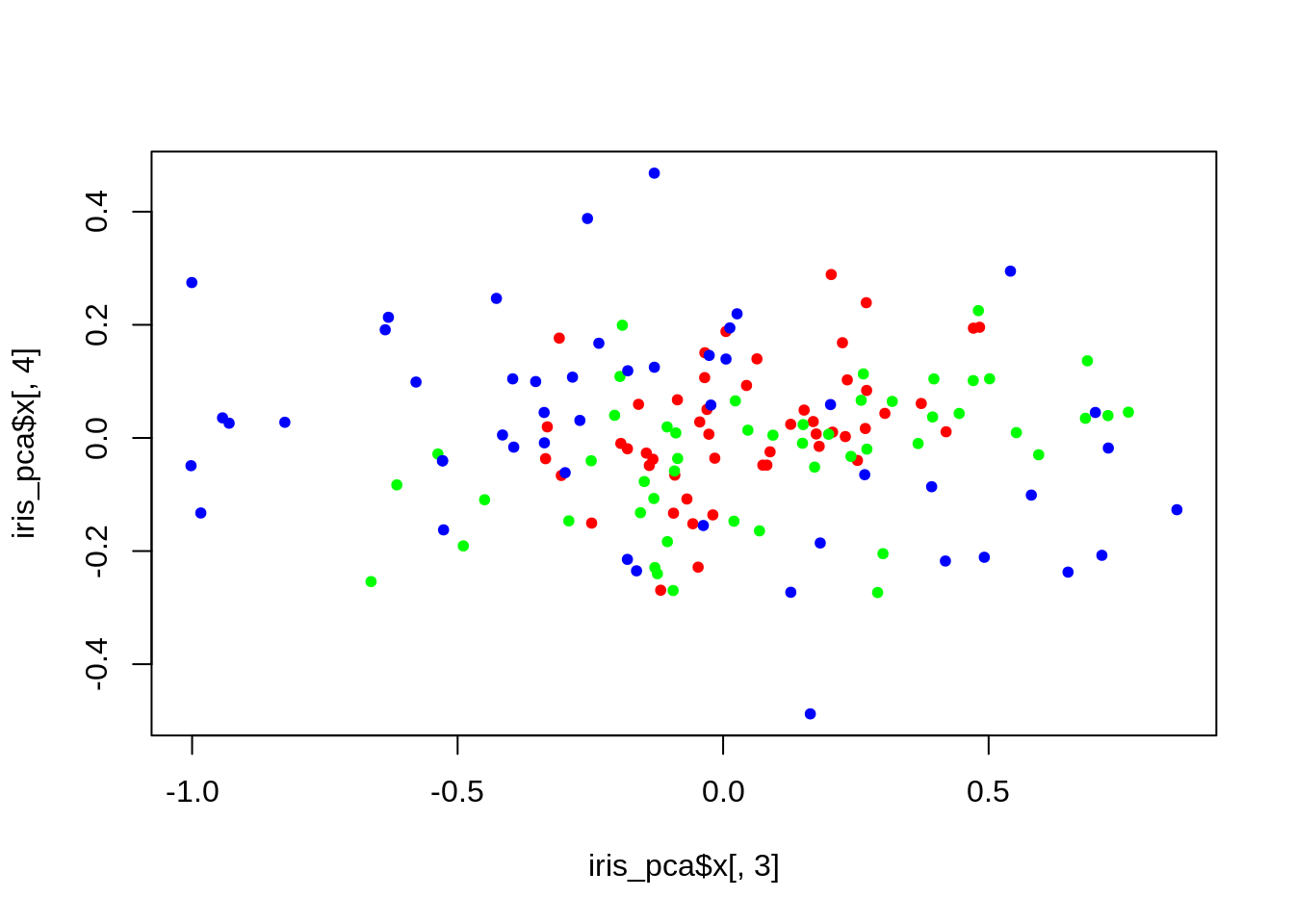iris_pve = get_PVE(iris_pca) plot( cumsum(iris_pve), xlab = "Principal Component", ylab = "Cumulative Proportion of Variance Explained", ylim = c(0, 1), type = 'b' )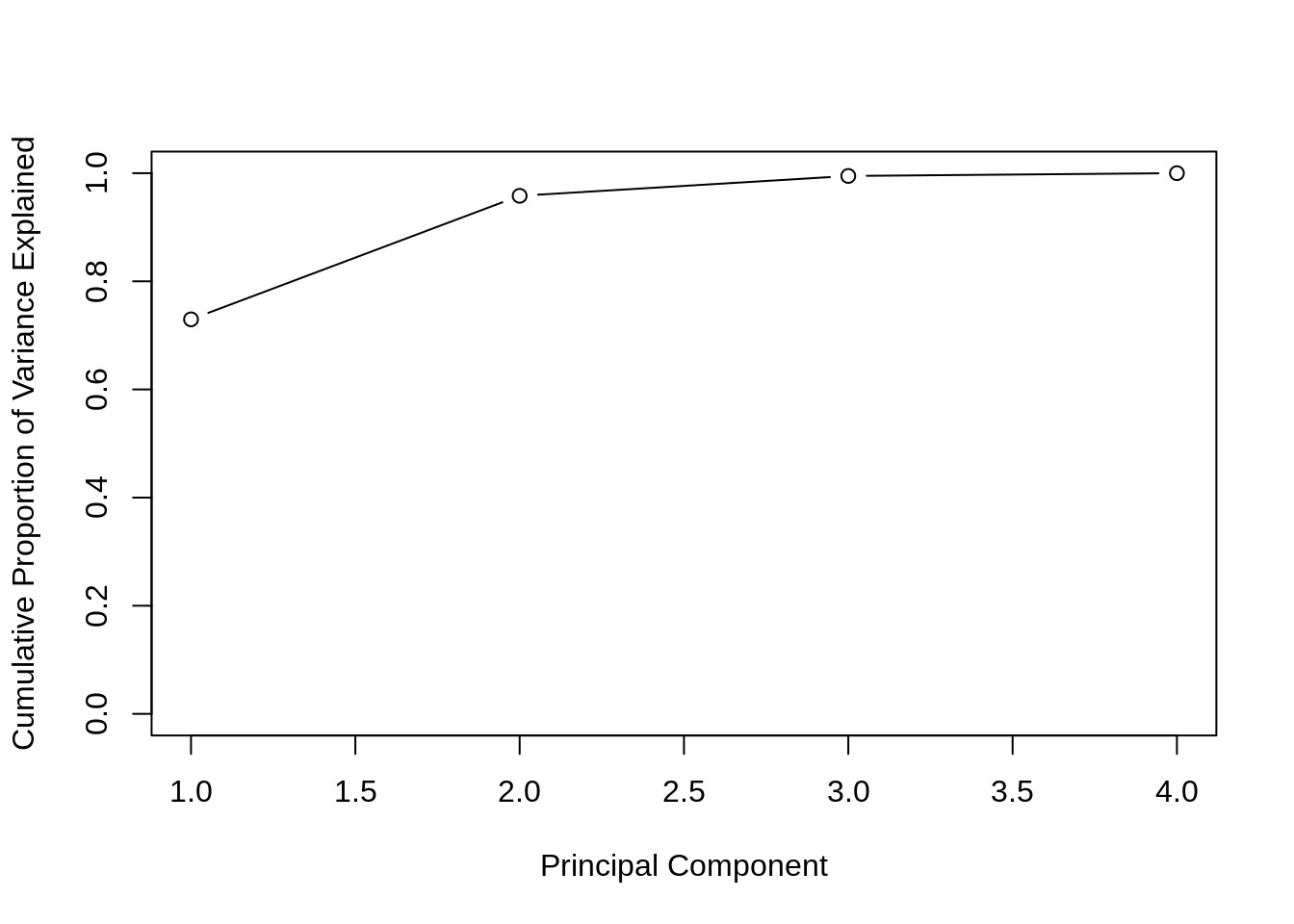iris_kmeans = kmeans(iris[,-5], centers = 3, nstart = 10) table(iris_kmeans$clust, iris[,5])
##
##     setosa versicolor virginica
##   1     50          0         0
##   2      0          2        36
##   3      0         48        14
iris_hc = hclust(dist(scale(iris[,-5])), method = "complete")
iris_cut = cutree(iris_hc , 3)
ColorDendrogram(iris_hc, y = iris_cut,
labels = names(iris_cut),
branchlength = 1.5)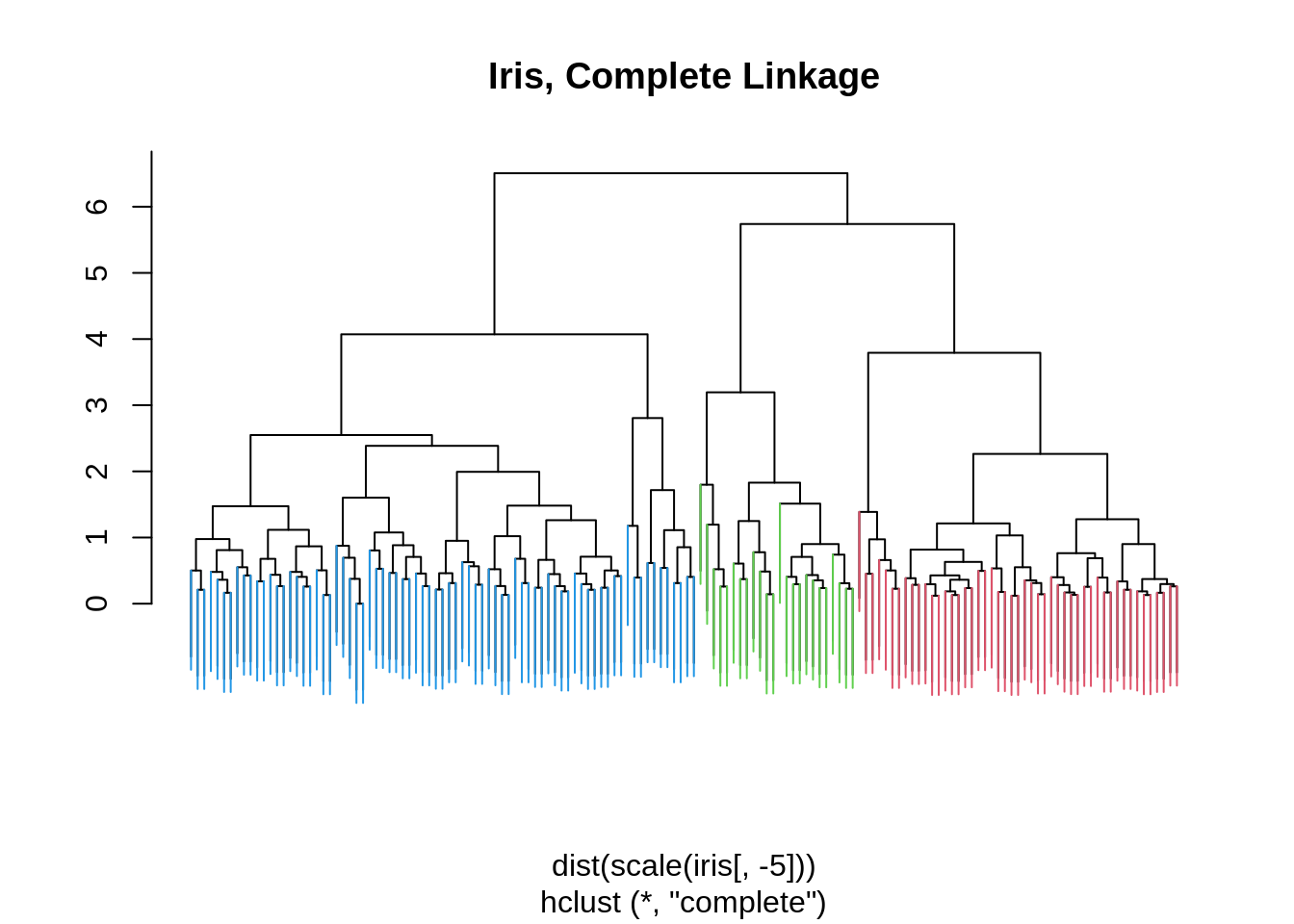table(iris_cut, iris[,5])
##
## iris_cut setosa versicolor virginica
##        1     49          0         0
##        2      1         21         2
##        3      0         29        48
table(iris_cut, iris_kmeans\$clust)
##
## iris_cut  1  2  3
##        1 49  0  0
##        2  1  0 23
##        3  0 38 39
iris_hc = hclust(dist(scale(iris[,-5])), method = "single")
iris_cut = cutree(iris_hc , 3)
ColorDendrogram(iris_hc, y = iris_cut,
labels = names(iris_cut),
branchlength = 0.3)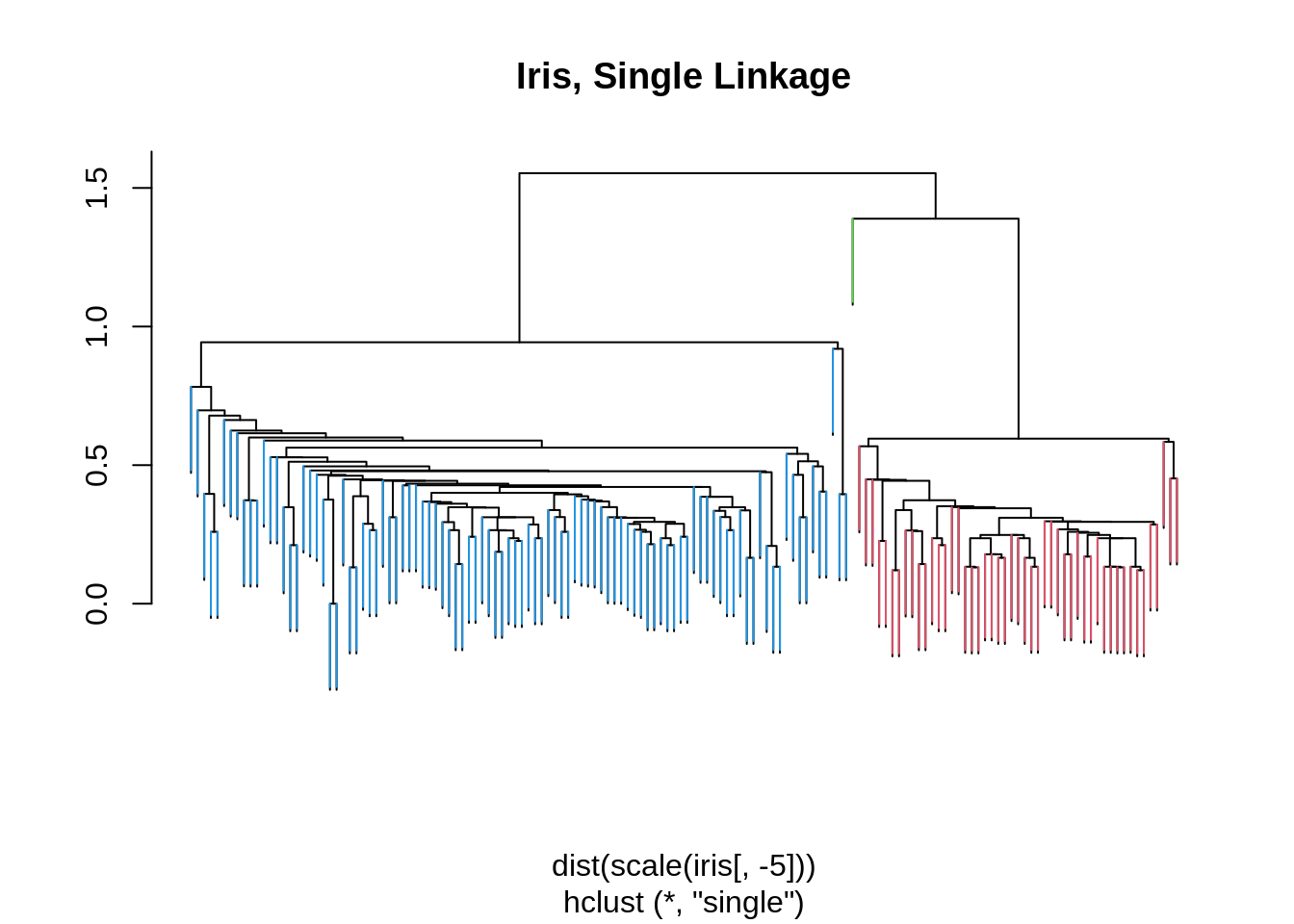iris_hc = hclust(dist(scale(iris[,-5])), method = "average")
iris_cut = cutree(iris_hc , 3)
ColorDendrogram(iris_hc, y = iris_cut,
labels = names(iris_cut),
branchlength = 1)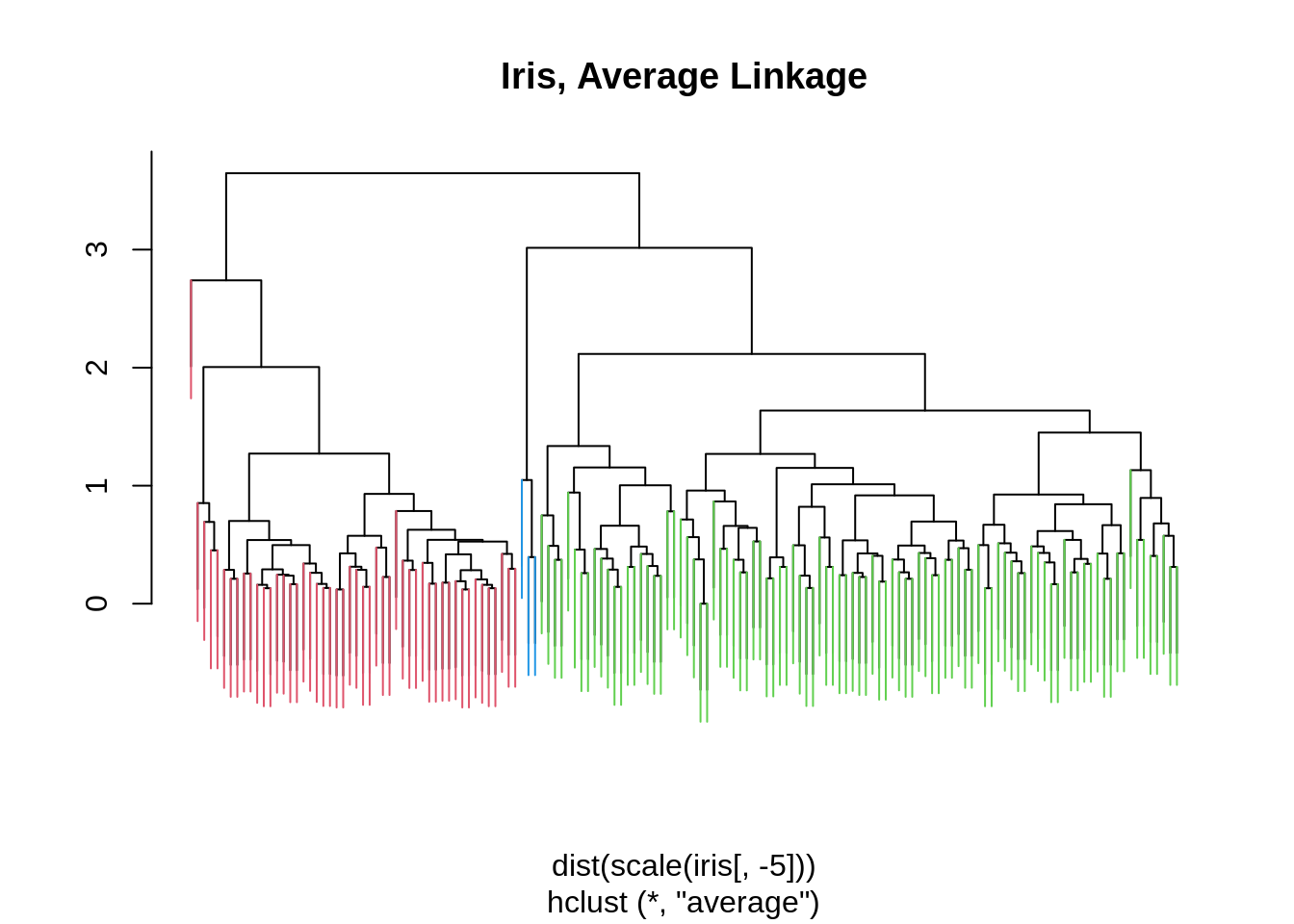## 13.4 RMarkdown

The RMarkdown file for this chapter can be found here. The file was created using R version 4.0.2 and the following packages:

• Base Packages, Attached
##  "stats"     "graphics"  "grDevices" "utils"     "datasets"  "methods"
##  "base"
##  "sparcl"  "MASS"    "mlbench" "caret"   "ggplot2" "lattice" "ISLR"
##   "tidyselect"   "xfun"         "purrr"        "reshape2"     "splines"
##  "nnet"         "nlme"         "compiler"